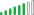cancel
Showing results for
Did you mean:Helper IV

## How to calculate % change over a custom period from last year?

How would you calculate the % change over the same months from this year to last year?

For example, I would like to calculate the % change from January to April of this year to January to April of last year?

I can't do YTD calculation or calculate using Quarters....any suggestions?

Please assume the date field is called ProjDate.

Thanks!

1 ACCEPTED SOLUTIONSolution Supplier

I assume you have a total sales measure

Try this

Total Sales Last Year = CALCULATE ( [Total Sales], SAMEPERIODLASTYEAR(YourDateTable[ProjDate]))

Diff This Year - Last Year = IF(ISBLANK([Total Sales Last Year]), BLANK(),[Total Sales] - [Total Sales Last Year])

% Diff = DIVIDE ([Diff This Year - Last Year],[Total Sales Last Year])Solution Supplier

I assume you have a total sales measure

Try this

Total Sales Last Year = CALCULATE ( [Total Sales], SAMEPERIODLASTYEAR(YourDateTable[ProjDate]))

Diff This Year - Last Year = IF(ISBLANK([Total Sales Last Year]), BLANK(),[Total Sales] - [Total Sales Last Year])

% Diff = DIVIDE ([Diff This Year - Last Year],[Total Sales Last Year])